# Resistor association

Resistors are devices that convert electrical energy into thermal energy. Through the association of resistors, we can obtain different values ​​of electrical resistance.

Resistors are devices capable of transforming electrical energy into thermal energy through the Joule effect . The electrons of the electric current , which pass through the resistor, collide with its atoms, producing an increase in its thermal agitation, consequently, the resistor emits energy in the form of heat to its surroundings.

When the electrons of the electric current pass through the resistor, they lose part of their energy, transferring it to the atoms of the crystal lattice of the material. In this process, we say that the electric voltage , measured in Volts (V) and the electric current, measured in Ampere (A), undergoes a decrease in its magnitude that is proportional to the electrical resistance of the material that makes up the resistor.

Resistors are produced in many different sizes and electrical resistances.

## Resistor association

As resistors are used for many different applications, they are produced in different modules of electrical resistance, however, it is likely that you will not find a resistor with a specific resistance, in this case, we can make different associations between resistors. When we associate two or more resistors, the resistance of the set becomes different from the individual resistances of each resistor.

According to the form of association we adopt, it is possible to greatly increase or decrease the value of the equivalent resistance of a circuit. Check out some ways of associating resistors:

## Combination of resistors in series

The association of resistors in series is done when we connect the resistors on the same branch (wire) of a circuit. When connected in series, all resistors carry the same electrical current The figure below shows a diagram of what three resistors (R 1 , R 2 and R 3 ) would be associated in series, check it out: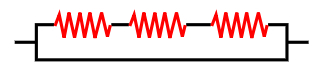In series association, the electric current is the same for all resistors.

To determine the equivalent resistance of this combination of resistors, we will apply Ohm’s 1st law to them . This law tells us that the total voltage drop in the circuit (U T ), measured in volts (V), is given by the sum of the individual voltage drops (U 1 , U 2 and U 3 ), caused by the resistances of each one of the resistors (R 1 , R 2 and R 3 ).

These individual voltage drops are given by the product of the electrical resistance (R) , measured in ohms (Ω) by the electrical current (i) through each resistor, measured in ampere (A):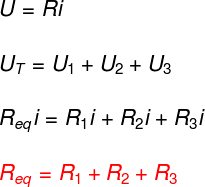In the equivalent resistance of series-associated resistors, we add the individual resistances.

A more general law can be written as a summation of all n resistors that are connected in series in the same branch of the circuit: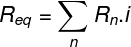Based on this law shown, we can see that the above association always increases the magnitude of the electrical resistance of a circuit.

## Connecting resistors in parallel

The association of resistors in parallel is done when two or more resistors are placed in different branches of a circuit, delimited by two consecutive nodes.When resistors are connected in parallel, all resistors in the association are connected to the same electrical potential.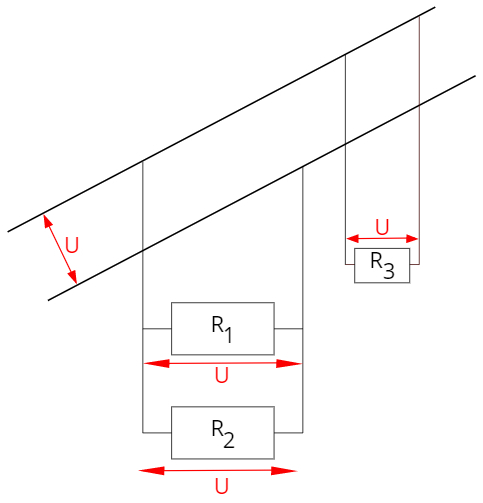In the figure above, resistors R 1 , R 2 and R 3 are connected in parallel and, therefore, they are under the same electrical voltage.

This type of association is used in residential electrical installations, where it is necessary that all electrical appliances operate under the same electrical voltage, for example. However, the greater the number of devices connected in series, the smaller the current flowing through each one must be.

Since the electrical voltage is the same for all resistors associated in parallel, the electrical currents in each resistor are different (if their resistances are different). In this way, the total electric current (i T ) is given by the sum of the electric currents that pass through each of the resistors (i 1 , i 2 and i 3 ), we can determine the equivalent resistance of the association in a similar way to what we did for the resistors in series – applying ohm’s 1st law, note: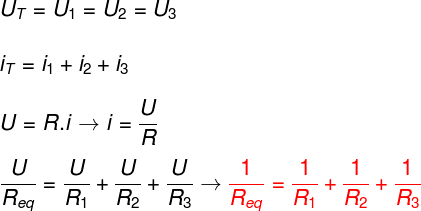We can write a more general law, able to calculate the equivalent resistance of n resistors associated in parallel, note: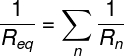In the particular case where there are only two resistors connected in parallel, we can also use a slightly simpler formula, given by the product of the resistances divided by their sum: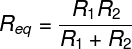Another particular case concerns identical resistors connected in parallel. In this case, we can divide the individual resistance by the number of resistors: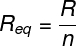Looking at the formulas given above, it is possible to notice that, in parallel resistance, the equivalent resistance will always be less than the smallest of the individual resistances.

## Mixed association of resistors

The mixed combination of resistors can be separated into parallel and series associations. Check out an example of a circuit formed by resistors in mixed association:

the two resistors on the upper branch are connected in series. The resistor equivalent to their association, in turn, is associated in parallel with the resistor of the lower branch.

## Resistor association solved exercises

1) Two resistors, R 1 and R 2 , of 0.5 Ω each are connected in series. In parallel with these resistors, there is a resistor (R 3 ) of 0.25 Ω . Determine the equivalent resistance of this combination of resistors.

Resolution:

First, we must calculate the resistance of resistors that are connected in series. For that, we just need to add their individual resistances. Then we calculate the equivalent resistance in parallel with the result obtained and with the resistance of 0.25 Ω, note: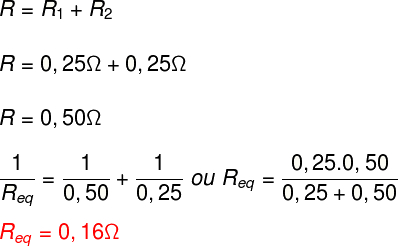two) An electrical circuit is formed by 500 resistors of 1.5 kΩ resistance connected in parallel. Determine the equivalent electrical resistance of this circuit. (k = kilo – 10³)

Resolution:

Since all the resistors in question are identical and connected in parallel, we can calculate the equivalent resistance of the circuit simply by dividing the value of the individual resistance by the number of resistors: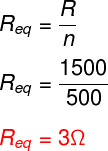Check Also
Close